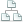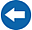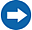Home >Tips >Excel 2016 from Scratch >Vertical lookup BSite map

# Excel 2016 from Scratch Exercise Vertical Lookup B

## An Excel tutorial by Peter KalmstromIn the previous exercise in the Excel 2016 from Scratch series, Peter Kalmstrom introduced formulas with the vertical lookup (VLOOKUP) function. Here is an exercise, that you can try yourself before you look at the solution in the demo.

As an example Peter uses a calculation of flooring with different materials in rooms of different sizes. The formula looks up the cost of the selected flooring to calculate the cost for each room.

To have a more detailed explanation of the vertical lookup function used in this exercise, please refer to the next article. It uses the same example exercise, but it does not calculate the floor area. It just explains how to use the function guide to create a formula with a vertical lookup.

### Exercise

If you want to try the exercise yourself, please download the Excel file Peter uses in the demo!

### Content

This is what Peter shows in the demo below:

• How to write a formula that calculates a rectangular area by multiplying its width and lenght.
• Brief explanation on how to use the function guide to create a formula with a vertical lookup. See next article for details.
Peter of course also shows how to check a formula, how to fill down a formula and other things that he has shown in several earlier demos too. These things need to be done almost every time!

Peter uses Excel 2016 for his demo, but the Excel calculation functions are the same for earlier versions of Excel.